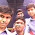### General Gas Equation

The gas laws give us the relation between the two variables when the third variable is constant. But in actual practice, all the three variables i.e.. pressure, volume and temperature, change simultaneously. In order to deal with all practical cases, the Boyle's law and Charles' law are combined together, which gives us a general gas equation.

According to Boyle's law ... (Keeping T constant)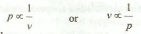and according to Charles' law ...(Keeping p constant)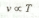It is obvious that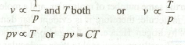where C is a constant, whose value depends upon the mass and properties of the gas concerned. The more useful form of the general gas equation is :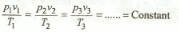where suffixes 1, 2 and 3 refer to different sets of conditions.

1.